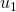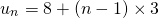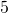# Arithmetic Sequences: Development

Let’s figure out a formal approach to arithmetic sequences.

In the applet below, you are given the first term, and the common difference. You are then asked to calculate one of the other terms of the sequence.

Notice the calculation that you are repeating each time. Let’s call the first termand the common difference. What calculation do you perform each time you wish to calculate

1.?
2.?
3.?

The answer to the third question is the a formula that may be used to calculate the general term for an arithmetic sequence.

## Example 1

An arithmetic sequence has first termand common difference. Find an expression for any term of the sequence,.

Solution

The first five terms of the sequence areWe know that. We can replacewithand we can replacewith.It’s helpful to put the 3 before the brackets:We can conclude that.

Now we can use this formula to find any term in the sequence: let’s find the fifth term. We replace the valuewith..

We can confirm this calculation with the sequence written out above.

## Example 2

An arithmetic sequence has first termand common difference. Find an expression for any term of the sequence,.

Solution

The first five terms of the sequence areWe know that. We can replacewithand we can replacewith.It’s helpful to put the (-3) before the brackets, remember to take the negative sign with you:We can conclude that.

Now we can use this formula to find any term in the sequence: let’s find the fourth term. We replace the valuewith..

We can confirm this calculation with the sequence written out above.

Find the general term for the following: